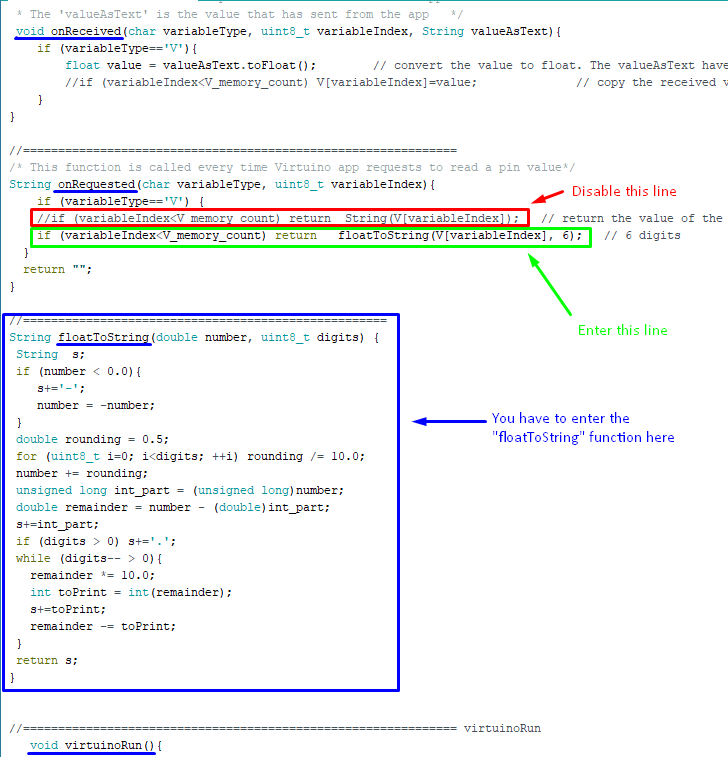How modify Virtuino library to support more than two decimal places

VirtuinoCM library

Step 1:

Open a sketch of the virtuinoCM library  examples
then put this function in the file

//====================================================
String floatToString(double number, uint8_t digits) {
String  s;
if (number < 0.0){
s+='-';
number = -number;
}
double rounding = 0.5;
for (uint8_t i=0; i<digits; ++i) rounding /= 10.0;
number += rounding;
unsigned long int_part = (unsigned long)number;
double remainder = number - (double)int_part;
s+=int_part;
if (digits > 0) s+='.';
while (digits-- > 0){
remainder *= 10.0;
int toPrint = int(remainder);
s+=toPrint;
remainder -= toPrint;
}
return s;
}

Step 2:

Make these changes to the code